numpy.diag()返回一个矩阵的对角线元素，或者创建一个对角阵（ diagonal array.）。我们还是用例子来说明问题：

• 先引入numpy• 创建一个对角阵，如下• 创建一个二维数组x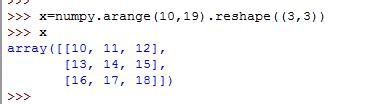• 提起二维数组的对角元素的值，构成一个一位数组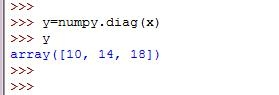• 或者，我们可以使用第二个参数，获取其他元素，请自行观察这个值在x数组中的位置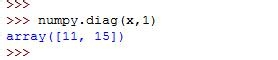• 接着，你换一个参数试试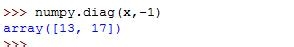• 连续使用两个diag，可以得到一个对角阵，除对角线以外的元素均为零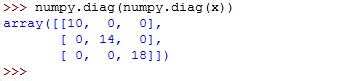• 今天用到的所有代码：

• numpy.diag((1,2,3))

• array([[1, 0, 0],

• [0, 2, 0],

• [0, 0, 3]])

• x=numpy.arange(9).reshape((3,3))

• x

• array([[0, 1, 2],

• [3, 4, 5],

• [6, 7, 8]])

• x=numpy.arange(10,19).reshape((3,3))

• x

• array([[10, 11, 12],

• [13, 14, 15],

• [16, 17, 18]])

• y=numpy.diag(x)

• y

• array([10, 14, 18])

• numpy.diag(x,1)

• array([11, 15])

• numpy.diag(x,-1)

• array([13, 17])

• numpy.diag(np.diag(x))

• Traceback (most recent call last):

• File “<pyshell#38>”, line 1, in

• numpy.diag(np.diag(x))

• NameError: name ‘np’ is not defined

• numpy.diag(numpy.diag(x))

• array([[10, 0, 0],

• [ 0, 14, 0],

• [ 0, 0, 18]])

### 赞助